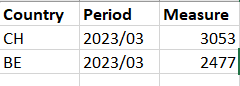Announcements
Applications are open for the 2024 Qlik Luminary Program. Apply by December 15 here.
cancel
Showing results for
Did you mean:Creator

## How to display previous month value not regarding current selection (YTD Table calculation) ?

Hi eveyrone,

i have this sheet where user can select a YearMonth date.
The problem is that i would like to show, regarding of the selection all the previous value for the measure, like a YTD calculation.

The measure i have setup is this, which excludes previous year selection, but mantain all the months of the year selected in the YearMonth dimension:

``````RangeSum(Above(total sum({<YearMonth= ,Year = p(Year) >} cod_connid_2_3_4_6_7_v), 0, RowNo()))
``````

Let me show with an example:

i have this table where i have selected yearmonth =  2023/03As you can see, the table, shows me all the date in the 2023.

What i want:

if i select YearMonth = 2023/03 i would like to show something like thisMoreover, period is a dynamic field where user can select Weekly, Date, and Month and it's based on a variable and Rangesum will not work with only one row.

I've tried also YTD flag and set expression, but they are not likely to work if i keep the time dimension in the report.

How can i achieve it?

Labels (3)

• ### Set Analysis

4 RepliesMVP

Try this

RangeSum(Above(total sum({<YearMonth={"<=\$(=Max(YearMonth))"}, Year = {\$(=Max(Year))}>} cod_connid_2_3_4_6_7_v), 0, RowNo()))Creator
Author

thank you!!

this one works, but it shows me even previous period like this:i would like to show only 2023/03 rowsMVP

Try this

RangeSum(Above(total sum({<YearMonth={"=\$(=Max(YearMonth))"}, Year = {\$(=Max(Year))}>} cod_connid_2_3_4_6_7_v), 0, RowNo()))

Or

sum({<YearMonth={"\$(=Max(YearMonth))"}, Year = {\$(=Max(Year))}>} cod_connid_2_3_4_6_7_v)Creator
Author

this one will not work, it's a set expression which take in account only the select dimension in the filter.Tags
Community Browser